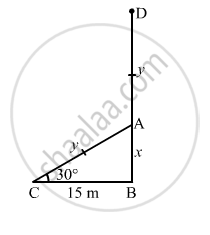Advertisement Remove all ads

# The Upper Part of a Tree Broken by the Wind Makes an Angle of 30° with the Ground and the Distance from the Root to the Point Where the Top of the Tree Touches the Ground is 15 M. Using Si - Mathematics

The upper part of a tree broken by the wind makes an angle of 30° with the ground and the distance from the root to the point where the top of the tree touches the ground is 15 m. Using sine rule, find the height of the tree.

Advertisement Remove all ads

#### Solution

Suppose BD be the tree and the upper part of the tree is broken over by the wind at point A.$\text{ The total height of the tree is x + y } .$
$\text{ In } \bigtriangleup ABC,$
$\angle C = 30° \text{ and } \angle B = 90° .$
$\therefore \angle A = 60° .$
$\text{ So, on using sine rule, we get }:$
$\frac{AB}{\sin30° } = \frac{BC}{\sin60° } = \frac{AC}{\sin90°}$
$\Rightarrow \frac{x}{\sin30°} = \frac{15}{\sin60°} = \frac{y}{\sin90°}$
$So, \frac{x}{\sin 30°} = \frac{15}{\sin60°}$
$\Rightarrow \frac{x}{\frac{1}{2}} = \frac{15}{\frac{\sqrt{3}}{2}}$
$\Rightarrow x = \frac{15}{\sqrt{3}} = 5\sqrt{3}$
$\text{ Also },$
$\frac{15}{\sin60° } = \frac{y}{\sin90°}$
$\Rightarrow \frac{15}{\frac{\sqrt{3}}{2}} = y$
$\Rightarrow y = \frac{30}{\sqrt{3}} = 10\sqrt{3}$
$\text{ So, the height of the tree is } x + y = 5\sqrt{3} + 10\sqrt{3} m$
$= 15\sqrt{3}m$

Concept: Sine and Cosine Formulae and Their Applications
Is there an error in this question or solution?
Advertisement Remove all ads

#### APPEARS IN

RD Sharma Class 11 Mathematics Textbook
Chapter 10 Sine and cosine formulae and their applications
Exercise 10.1 | Q 28 | Page 14
Advertisement Remove all ads
Advertisement Remove all ads
Share
Notifications

View all notifications

Forgot password?